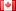# Revision history [back]

### solve x*exp(x)==0 and x*exp(-x)==0

sage: solve(x*exp(x)==0,x) [x == 0, e^x == 0]

but

sage: solve(x*exp(-x)==0,x) [x == 0]

How can i avoid the e^x==0 in the solutionlist?

Thanks for help.2 No.2 RevisionDSM5242 ●21 ●68 ●112

### solve x*exp(x)==0 and x*exp(-x)==0

sage: solve(x*exp(x)==0,x)
[x == 0, e^x == 0]0]


but

sage: solve(x*exp(-x)==0,x)
[x == 0]0]


How can i avoid the e^x==0 in the solutionlist?

Thanks for help.